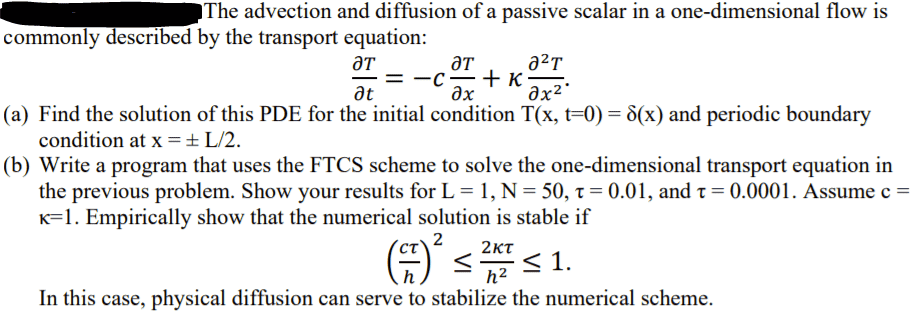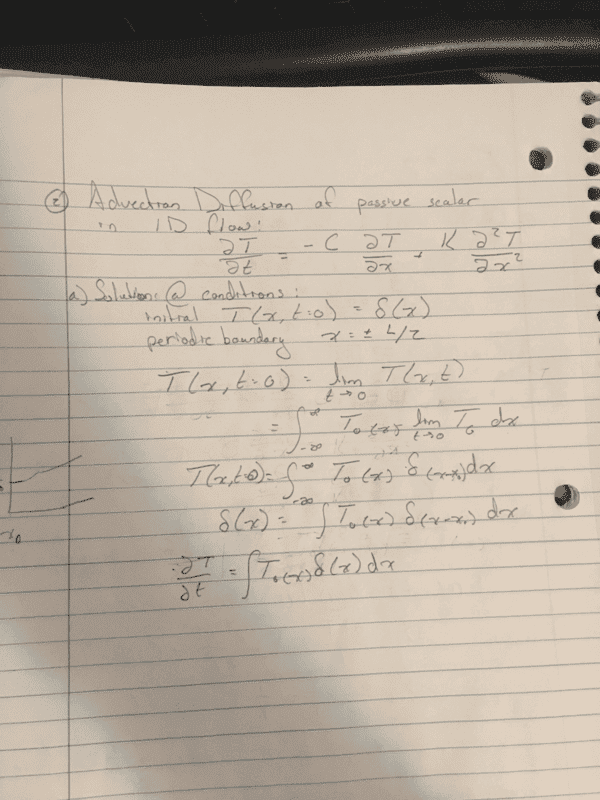jkthejetplane
Homework Statement:
Solving PDE with ainitial conditions
Relevant Equations:
I have no idea where to go wit this i started following something we did in class before but it doesnt quite make sense. I could really use help. I think i will be able to get the program if i can figure out these solutions
ThanksMentor
I'm having trouble seeing what you did in your handwritten analysis. Can you first tell us your rationale for solving this? That periodic BC seems very peculiar. What does it even mean?

jkthejetplane
I'm having trouble seeing what you did in your handwritten analysis. Can you first tell us your rationale for solving this? That periodic BC seems very peculiar. What does it even mean?
Yeah I actually don't know what i was doing haha
I started following our notes on something similar and got completely lost. My attempt has basically no merit. The boundary conditions were given by the problem

William Crawford
Your PDE is linear, you should therefore try to look for a solution by separation of variables; i.e. assume that the solution is of the form ##T(t,x) = u(t)v(x)## and derive a space-independent equation for ##u(t)## and a time-independent equation for ##v(x)##.

Remember that ##v(x)## inherit the periodic boundary condition from ##T##.

Last edited:
Mentor
I would definitely not use separation of variables in obtaining an analytic solution to this problem. Here is the approach I would recommend:

1. Start with the analytic solution for the case of C = 0, and on the infinite x interval from minus infinity to plus infinity. This is a closed-form single term expression (not a series of sines and cosines).

2. Add the periodicity to this solution by putting reflective boundary conditions at x = L/2 and x =-L/2 by taking a superposition of terms in the form of 1. , based on delta functions being initially located not only at x = 0, but also at x = + nL and x = - nL, with n = 1,2,3,...

3. Add the translation (advection) by substituting x - Ct for x in all the terms of 2.

This will satisfy the differential equation, the initial condition, and the boundary conditions